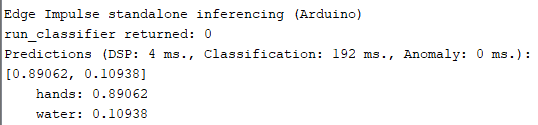# Completely different classification on device and on live classification

I’ve trained a model and deployed it on an Arduino Nano using the arduino library. I’m getting completely different classifications when I used the static buffer example with features from the live classification. The expected result was class 48.

Hi @evan !

Could you share me your project ID if you don’t mind me to join your project to test it.

Best,

Louis

I’m not sure if this is the project ID or not: Evan / evan-project-1 v4

Hi @evan.

Well… I’m having the same issue:

when it should be:Investigating…

1 Like

Hi, I’m having the same problem. In the static_buffer example, copying the raw features even for different images results in the same classification of 0.89602. The expected result is water.I’ve also tried copying the raw data from the .txt files I created to be converted to .jpg using the `framebuffer2jpg` tool. I’m deploying on an Arduino Nano 33 BLE Sense as well.

Project ID: 24610
Arduino core version: 1.3.2

@evan Interesting, I see a discrepancy between the features generated in the Studio and in the C++ Library. Investigating now.

edit: So there’s a bug when setting low frequency to `0`. If you set it to `300` you can work around this. I’ll file a bug for the SDK team. The Python implementation (which we use underneath for the Studio defaults to 300 if set to 0, while the SDK just sets it to 0, we’ll push a fix).

2 Likes

@s-agar might better to open a new post, but you found a very interesting bug! For quantized neural networks where the input is an image we save memory by directly quantizing data from the `signal_t` structure into the memory managed by the neural network (rather than using intermediate buffers as we do for other DSP blocks). There’s a few clever tricks here to do this as fast as possible, but there was an assumption that the scaling parameters of the input layer (how inputs map to their quantized counterpart) are always the same (scaling value of `1/255` - which is virtually always what the quantizer uses, as pixels are ranged from `0..255` and the input layer of the NN has `int8` inputs - easy).

Now the interesting bit… Because all your images are so dark (range of pixel values after turning your image into grayscale are around `0..30`), the neural network chooses different quantization parameters, and thus the assumptions when directly quantizing break… We’re pushing a fix on Monday, but you can patch the `extract_image_features_quantized` function in `ei_run_dsp.h` like this as a quick workaround:

``````__attribute__((unused)) int extract_image_features_quantized(signal_t *signal, matrix_i8_t *output_matrix, void *config_ptr, const float frequency) {
ei_dsp_config_image_t config = *((ei_dsp_config_image_t*)config_ptr);

int16_t channel_count = strcmp(config.channels, "Grayscale") == 0 ? 1 : 3;

if (output_matrix->rows * output_matrix->cols != static_cast<uint32_t>(EI_CLASSIFIER_INPUT_WIDTH * EI_CLASSIFIER_INPUT_HEIGHT * channel_count)) {
ei_printf("out_matrix = %hu items\n", output_matrix->rows, output_matrix->cols);
ei_printf("calculated size = %hu items\n", static_cast<uint32_t>(EI_CLASSIFIER_INPUT_WIDTH * EI_CLASSIFIER_INPUT_HEIGHT * channel_count));
EIDSP_ERR(EIDSP_MATRIX_SIZE_MISMATCH);
}

size_t output_ix = 0;

#if defined(EI_DSP_IMAGE_BUFFER_STATIC_SIZE)
const size_t page_size = EI_DSP_IMAGE_BUFFER_STATIC_SIZE;
#else
const size_t page_size = 1024;
#endif

// buffered read from the signal
size_t bytes_left = signal->total_length;
for (size_t ix = 0; ix < signal->total_length; ix += page_size) {
size_t elements_to_read = bytes_left > page_size ? page_size : bytes_left;

#if defined(EI_DSP_IMAGE_BUFFER_STATIC_SIZE)
#else
#endif
if (!input_matrix.buffer) {
EIDSP_ERR(EIDSP_OUT_OF_MEM);
}

for (size_t jx = 0; jx < elements_to_read; jx++) {
uint32_t pixel = static_cast<uint32_t>(input_matrix.buffer[jx]);

float r = static_cast<float>(pixel >> 16 & 0xff) / 255.0f;
float g = static_cast<float>(pixel >> 8 & 0xff) / 255.0f;
float b = static_cast<float>(pixel & 0xff) / 255.0f;

if (channel_count == 3) {
output_matrix->buffer[output_ix++] = static_cast<int8_t>(round(r / EI_CLASSIFIER_TFLITE_INPUT_SCALE) + EI_CLASSIFIER_TFLITE_INPUT_ZEROPOINT);
output_matrix->buffer[output_ix++] = static_cast<int8_t>(round(g / EI_CLASSIFIER_TFLITE_INPUT_SCALE) + EI_CLASSIFIER_TFLITE_INPUT_ZEROPOINT);
output_matrix->buffer[output_ix++] = static_cast<int8_t>(round(b / EI_CLASSIFIER_TFLITE_INPUT_SCALE) + EI_CLASSIFIER_TFLITE_INPUT_ZEROPOINT);
}
else {
// ITU-R 601-2 luma transform
float v = (0.299f * r) + (0.587f * g) + (0.114f * b);
output_matrix->buffer[output_ix++] = static_cast<int8_t>(round(v / EI_CLASSIFIER_TFLITE_INPUT_SCALE) + EI_CLASSIFIER_TFLITE_INPUT_ZEROPOINT);
}
}

}

return EIDSP_OK;
}
``````
1 Like

Haha sorry for barging in on your post @evan. Thanks @janjongboom, this fixed the problem!

Thank you so much! It’s working well now. In the future would I be able to classify sounds with fundamental frequencies below 300Hz with MFE or will the low frequency still default to 300 for the studio and SDK?

@evan I’ve now patched up the release in the SDK, so it’s still dubious whether that’s correct. But set it to `1Hz` for now.

2 Likes